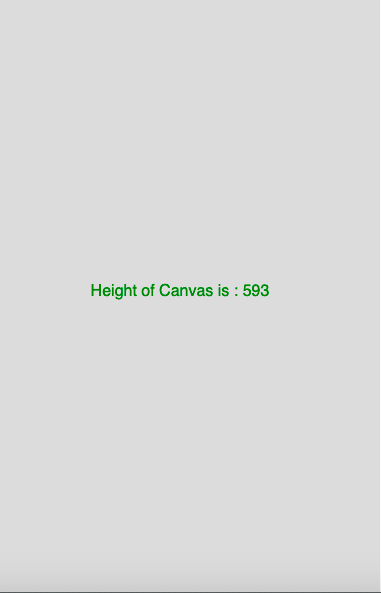# p5.js | height variable

• Last Updated : 16 Apr, 2019

The height variable in p5.js is a system variable which stores the height of the drawing canvas. It sets the second parameter of createCanvas() function.

Syntax:

Hey geek! The constant emerging technologies in the world of web development always keeps the excitement for this subject through the roof. But before you tackle the big projects, we suggest you start by learning the basics. Kickstart your web development journey by learning JS concepts with our JavaScript Course. Now at it's lowest price ever!

`height`

Below programs illustrate the height variable in p5.js:

Example 1: This example uses height variable to display the height of canvas.

 `function` `setup() {``     ` `    ``// Create Canvas of size 380*80  ``    ``createCanvas(380, 80);``}``  ` `function` `draw() {``     ` `    ``// Set the background color``    ``background(220);``     ` `    ``// Set the text size``    ``textSize(16);``     ` `    ``// Set the text alignment``    ``textAlign(CENTER);``     ` `    ``// Set the text color``    ``fill(color(``'Green'``));``     ` `    ``// Display result``    ``text(``"Height of Canvas is : "``        ``+ height, 180, 50);``} `

Output:Example 2: This example uses height variable to display the height of window.

 `function` `setup() {``  ``// set height to window height ``height = windowHeight;``  ``//create Canvas of size 380*80 ``  ``createCanvas(380, height);``}`` ` `function` `draw() {``  ``background(220);``  ``textSize(16);``  ``textAlign(CENTER);``  ``fill(color(``'Green'``));``  ``text(``"Height of Canvas is : "``+height, 180, height/2); ``//use of height variable``}`

Output:Reference: https://p5js.org/reference/#/p5/height

My Personal Notes arrow_drop_up